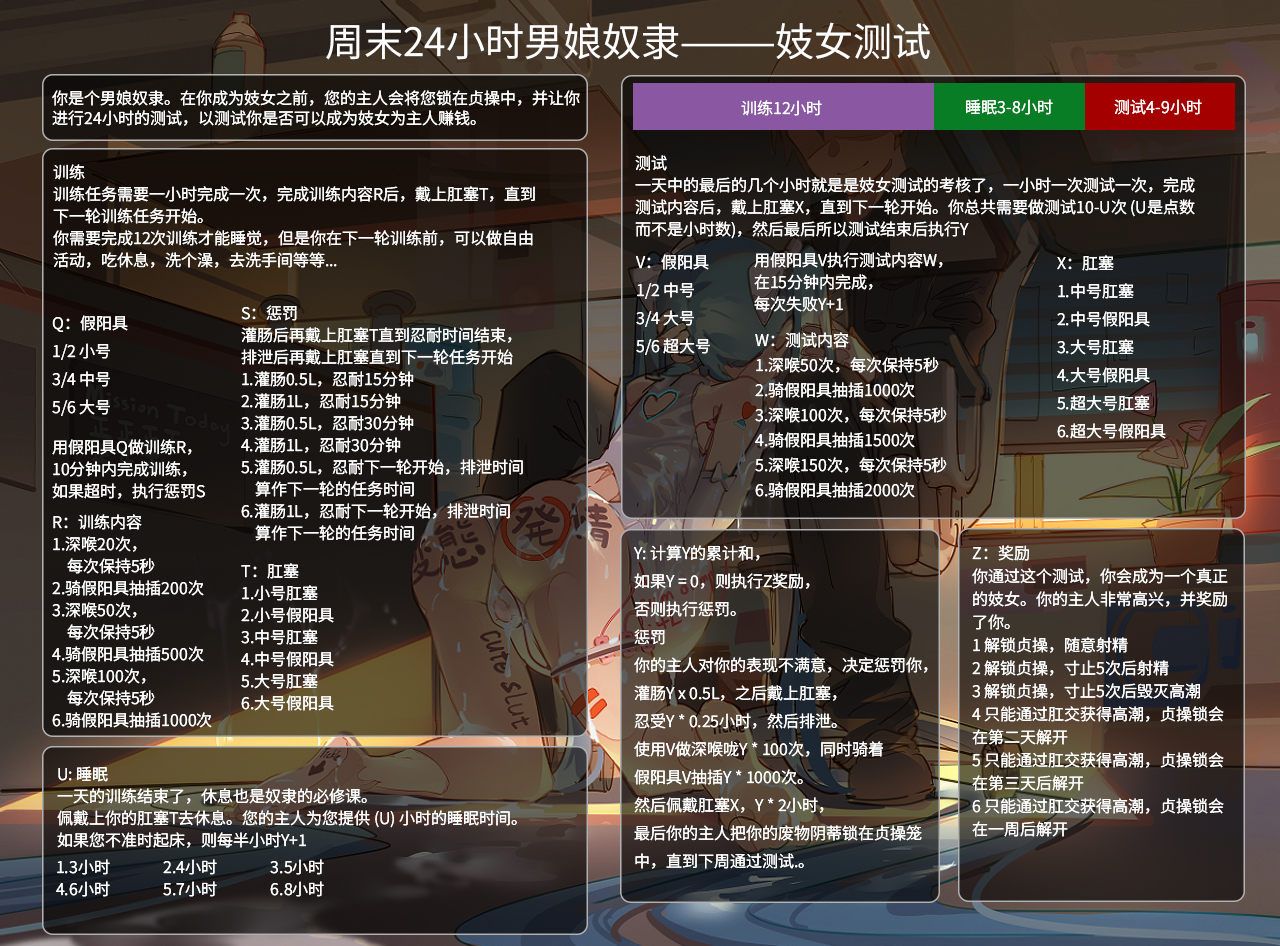Q=5 R=5 S=5 T=6 U=2 V=2 W=6 X=6 Y=1 Z=2